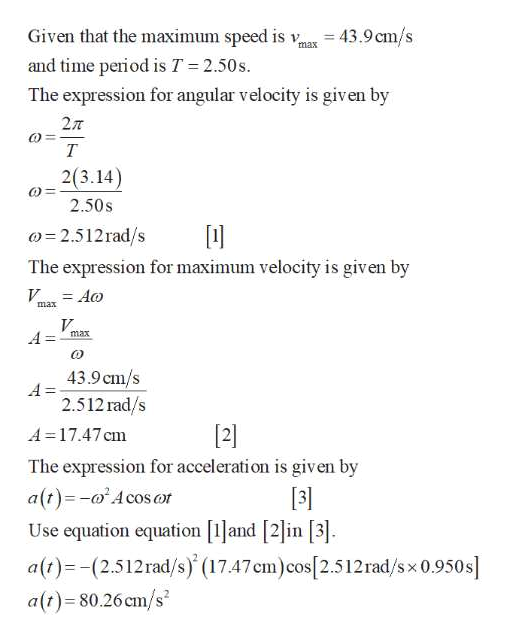# An air-track glider is attached to a spring on a frictionless horizontal surface. The air-track glider is pulled to the right and released from rest at t = 0 s. If the air-track glider reaches a maximum speed of 43.9 cm/s and oscillates with a period of 2.50 s, what is the air-track glider's acceleration at t = 0.950 s?a. 80.6 cm/s2b. -46.2 cm/s2c. -4.32 cm/s2d. 14.1 cm/s2

Question

An air-track glider is attached to a spring on a frictionless horizontal surface. The air-track glider is pulled to the right and released from rest at t = 0 s. If the air-track glider reaches a maximum speed of 43.9 cm/s and oscillates with a period of 2.50 s, what is the air-track glider's acceleration at t = 0.950 s?

a. 80.6 cm/s2
b. -46.2 cm/s2
c. -4.32 cm/s2
d. 14.1 cm/s2

check_circle

Step 1help_outlineImage TranscriptioncloseGiven that the maximum speed is v 43.9cm/s max and time period is T = 2.50s. The expression for angular velocity is given by 27T т 2(3.14) 2.50s 2.512rad/s The expression for maximum velocity is given by V Ao max V A= max 43.9 cm/s A= 2.512rad/s A=17.47 cm  The expression for acceleration is given by a(t)-Acosor Use equation equation [1and in . a(t)(2.512rad/s) (17.47cm)cos[2.512rad/s x 0.950s a(t) 80.26 cm/s fullscreen

### Want to see the full answer?

See Solution

#### Want to see this answer and more?

Solutions are written by subject experts who are available 24/7. Questions are typically answered within 1 hour.*

See Solution
*Response times may vary by subject and question.
Tagged in

### Other